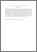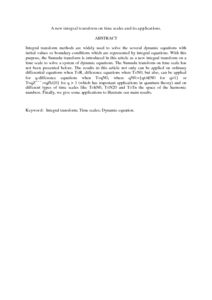Simple Search:

# A new integral transform on time scales and its applications.

## Citation

Ahmed Agwa, Hassan and Mohamed Ali, Fatma and Kilicman, Adem (2012) A new integral transform on time scales and its applications. Advances in Difference Equations, 2012 (60). pp. 1-26. ISSN 1687-1839

## Abstract / Synopsis

Integral transform methods are widely used to solve the several dynamic equations with initial values or boundary conditions which are represented by integral equations. With this purpose, the Sumudu transform is introduced in this article as a new integral transform on a time scale to solve a system of dynamic equations. The Sumudu transform on time scale has not been presented before. The results in this article not only can be applied on ordinary differential equations when T=R, difference equations when T=N0, but also, can be applied for q-difference equations when T=qN0, where qN0:={qt:t∈N0 for q>1} or T=qZ¯¯¯:=qZ∪{0} for q > 1 (which has important applications in quantum theory) and on different types of time scales like T=hN0, T=N20 and T=Tn the space of the harmonic numbers. Finally, we give some applications to illustrate our main results.Preview
PDF (Abstract)
A new integral transform on time scales and its applications.pdfView Item